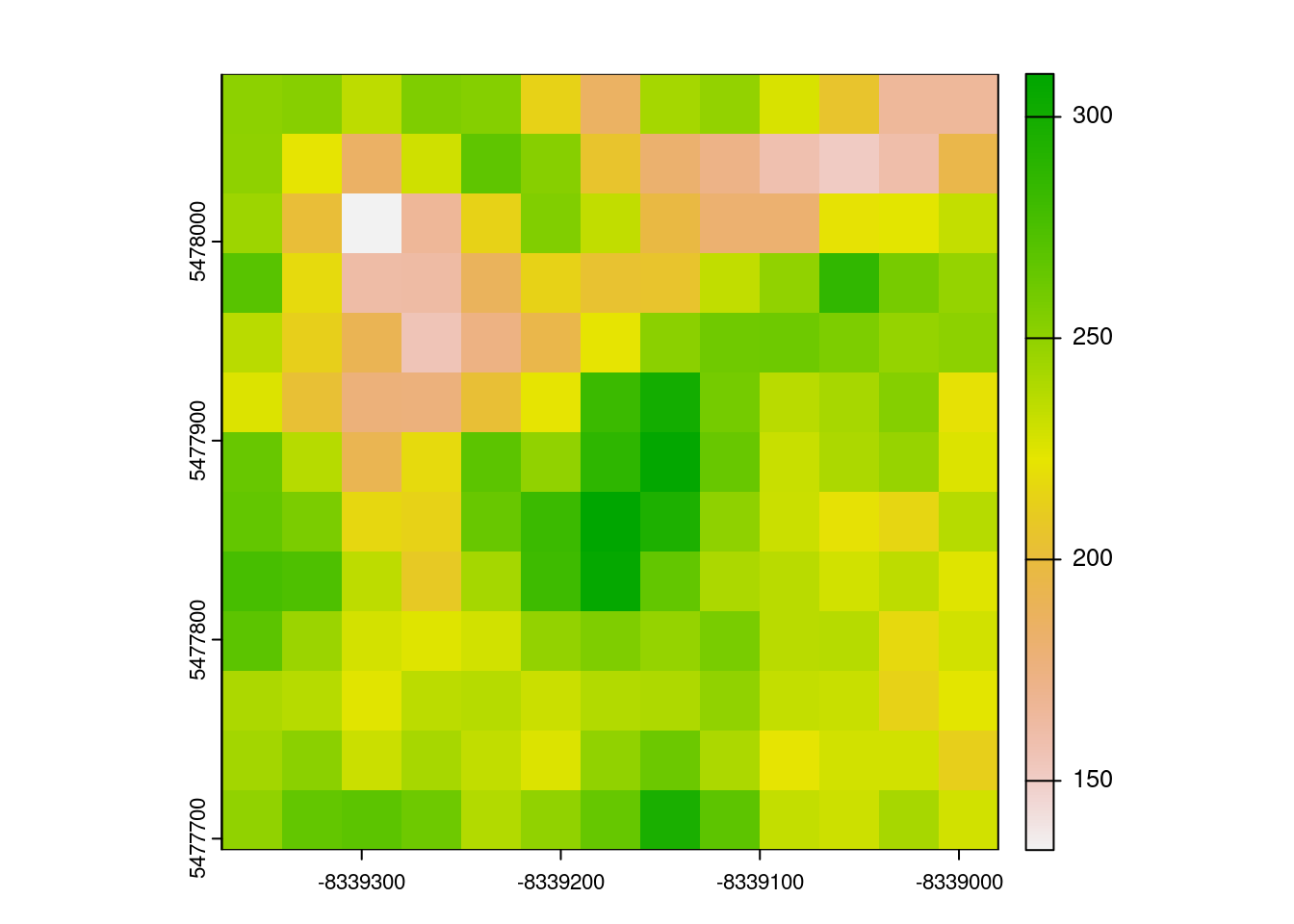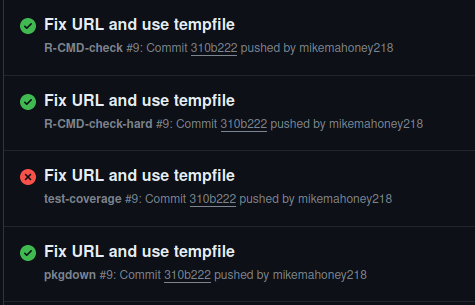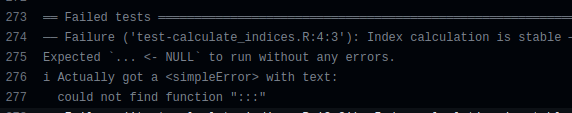# Executing R code from untrusted sources in minimal environments

Security improvements, if not fixes, by restricting what potentially-malicious code gets access to.
R
Tutorials
Author
Published

October 27, 2023

Yesterday we released rsi, an R package that (among other things) makes it easy to retrieve spectral indices from the Awesome Spectral Indices project and calculate them against any images you have on hand.

The actual code required to do these calculations is mostly just a bit of glue. The ASI project provides the formulas you’d use to calculate any spectral indices you might be interested in, which as relatively simple arithmetic are easily transformed into R code via `str2lang()`.

Once we’ve done that transformation, we can evaluate that R code against our images through a slightly off-label use of `terra::predict()`. This function loads chunks of our raster into R as a data frame with column names corresponding to our band labels, meaning that if we’re careful to ensure that our band names align with the standardized band names used by ASI1 we can compute indices by evaluating their formulas “with” the data frame.

This sounds complex, but doesn’t actually require that much code to put together:

``````calculate_indices <- function(raster,
indices,
output_filename) {
if (!inherits(raster, "SpatRaster")) raster <- terra::rast(raster)
formulas <- lapply(indices[["formula"]], str2lang)
terra::predict(
raster,
list(),
fun = function(model, newdata) {
out <- lapply(formulas, \(calc) with(newdata, eval(calc)))
names(out) <- indices[["short_name"]]
out
},
filename = output_filename
)
output_filename
}``````

Turn our formulas into calls, load our raster into R chunk by chunk, evaluate those calls in the context of our raster, lather rinse repeat. The conceptual complexity here is a lot higher, in my view, than the code complexity.

Because this code is pretty straightforward, and relies on the fantastic, highly-optimized terra package to actually do these computations, we’re able to calculate these indices fast:

``````example_index <- rsi::spectral_indices()
example_index <- example_index[example_index\$short_name == "DPDD", ]

system.time({
out <- calculate_indices(
system.file("rasters/example_sentinel1.tif", package = "rsi"),
example_index,
tempfile(fileext = ".tif")
)
})``````
``````   user  system elapsed
1.754   0.040   1.794 ``````
``terra::plot(terra::rast(out))``This is pretty nifty!

That said, we should be careful about what text we’re willing to turn into R code and execute. In particular, rsi is designed to integrate nicely with the Awesome Spectral Indices project, and to retrieve and compute the ASI set of indices – which, phrased differently, means we’re downloading code from the internet and running it on our computers. If someone were to mess with our indices – either by corrupting the GitHub repository or by editing the cached file on your machine – this could wind up giving them access to `system()` or other scary commands:

``````evil_index <- example_index
evil_index\$formula <- "system('echo oh no > /tmp/example.txt')"

try(
calculate_indices(
system.file("rasters/example_sentinel1.tif", package = "rsi"),
evil_index,
tempfile(fileext = ".tif")
),
silent = TRUE
)

`` "oh no"``

So, how can we make this safer?

One way is by taking away the number of toys any malicious code has available to play with. We can do this by running the code in a locked-down environment, where it won’t have access to functions that might let code mess with our machine.

One way of creating a locked down environment is `rlang::new_environment()`. By default, this function creates a new environment with nothing in it – no built-in functions or objects:

``ls(envir = rlang::new_environment())``
``character(0)``

This environment is also going to have the empty environment as its parent, meaning that code executed in this scope won’t be able to use functions or objects from the global environment2:

``````# inheriting from the global environment
local(
2 + 2,
new.env()
)``````
`` 4``
``````# inheriting from the empty environment
try(
local(
2 + 2,
rlang::new_environment()
)
)``````
``Error in 2 + 2 : could not find function "+"``

That means that any code we run inside of this new environment will only have access to whatever functions and variables we purposefully include in the environment. The `data` argument to `rlang::new_environment()` makes it relatively easy to define whatever objects we’re looking to make available in this new environment:

``local(2 + 2, rlang::new_environment(list(`+` = `+`)))``
`` 4``

That means that, if we create a minimal environment containing only the functions and variables essential for calculating our indices, we should hopefully be able to reduce the potential blast radius of malicious code – or at least make it a lot harder for malicious code to impact anything we care about. In rsi, that means we wind up calculating indices inside a minimal environment that looks like this:

``````calculate_indices <- function(raster,
indices,
output_filename) {
if (!inherits(raster, "SpatRaster")) raster <- terra::rast(raster)
formulas <- lapply(indices[["formula"]], str2lang)

exec_env <- rlang::new_environment(
list(
`::` = `::`,
`-` = `-`,
`(` = `(`,
`*` = `*`,
`/` = `/`,
`^` = `^`,
`+` = `+`,
`<-` = `<-`,
`{` = `{`,
`names<-` = `names<-`,
`function` = `function`,
list = list,
lapply = lapply,
with = with,
eval = eval,
formulas = formulas,
short_names = indices[["short_name"]],
raster = raster,
output_filename = output_filename
)
)

local(
{
terra::predict(
raster,
list(),
fun = function(model, newdata) {
out <- lapply(
formulas,
function(calc) {
with(newdata, eval(calc))
}
)
names(out) <- short_names
out
},
filename = output_filename
)
},
envir = exec_env
)

output_filename
}``````

This shouldn’t impact anything from the user’s perspective when calculating well-behaved formulas:

``````system.time({
out <- calculate_indices(
system.file("rasters/example_sentinel1.tif", package = "rsi"),
example_index,
tempfile(fileext = ".tif")
)
})``````
``````   user  system elapsed
0.016   0.000   0.015 ``````
``terra::plot(terra::rast(out))``But it makes the most obvious malicious code fail:

``````try(
calculate_indices(
system.file("rasters/example_sentinel1.tif", package = "rsi"),
evil_index,
tempfile(fileext = ".tif")
)
)``````
``````Error in system("echo oh no > /tmp/example.txt") :
could not find function "system"``````

Update 2023-10-27: However, we actually need to go one step further. Because we’ve included `::` in our minimal environment, we’ve left in an “escape hatch” that malicious code can use to access any functions it wants:

``````evil_index\$formula <- "base::system('echo oh no > /tmp/example2.txt')"
try(
calculate_indices(
system.file("rasters/example_sentinel1.tif", package = "rsi"),
evil_index,
tempfile(fileext = ".tif")
)
)``````
``Error : [predict] the number of values returned by 'fun' (model predict function) does not match the input. Try na.rm=TRUE?``
``readLines("/tmp/example2.txt")``
`` "oh no"``

Instead of calling `terra::predict()` via `::` inside our environment, we’ll need to include that function in the environment directly, in order to remove this escape hatch:

``````calculate_indices <- function(raster,
indices,
output_filename) {
if (!inherits(raster, "SpatRaster")) raster <- terra::rast(raster)
formulas <- lapply(indices[["formula"]], str2lang)

exec_env <- rlang::new_environment(
list(
`-` = `-`,
`(` = `(`,
`*` = `*`,
`/` = `/`,
`^` = `^`,
`+` = `+`,
`<-` = `<-`,
`{` = `{`,
`names<-` = `names<-`,
`function` = `function`,
list = list,
lapply = lapply,
with = with,
eval = eval,
formulas = formulas,
short_names = indices[["short_name"]],
raster = raster,
output_filename = output_filename,
predict = terra::predict
)
)

local(
{
predict(
raster,
list(),
fun = function(model, newdata) {
out <- lapply(
formulas,
function(calc) {
with(newdata, eval(calc))
}
)
names(out) <- short_names
out
},
filename = output_filename
)
},
envir = exec_env
)

output_filename
}``````
``````try(
calculate_indices(
system.file("rasters/example_sentinel1.tif", package = "rsi"),
evil_index,
tempfile(fileext = ".tif")
)
)``````
``Error in base::system : could not find function "::"``

Thanks Gábor Csárdi for the catch!

This still isn’t a perfect fix – and rsi also checks to make sure that all of your formulas are only using symbols that match the band names of your rasters. Even with these checks, you should investigate the formulas you’re going to run before you actually run them – or save a copy of the trusted indices you’re going to calculate and provide those to `calculate_indices()`, rather than using `spectral_indices()` directly. But this hopefully makes this function a pinch safer.

I wrote this piece of rsi back in August and then more or less didn’t think of it again until yesterday, when we released rsi on GitHub – and at the same time, started using GitHub Actions for CI for the package. All of a sudden, I started seeing a lot of CI runs that looked like this:R CMD check succeeding and test coverage failing made no sense to me, as theoretically they’re both running a full check and reporting the results. In classic developer tradition, I spent a few hours flailing around before finally giving up and resorting to the final option I had available: reading the error messages.

And it turned out that each of those failed test coverage runs had the same error message:`could not find function ":::"`.

My test coverage workflow is using the (fantastic) covr package to measure line coverage. Behind the scenes, covr is doing a lot more than just running R CMD check, like my other workflows – covr is actually changing what R runs when it runs your code, in order to measure how many times any given line of code gets called. This is a really neat workflow, but it doesn’t play nicely with our minimal environment here; the new code added inside of our `local()` statement depends upon functions that we didn’t (and aren’t going to) provide to the minimal environment, such as `:::`. Adding a new environment variable to the test coverage workflow, and skipping tests that ran the `local()` call when that environment variable was defined, wound up solving the issue.

## Footnotes

1. Which rsi will automatically enforce when using `get_*_imagery()` functions.↩︎

2. Or any other environments this one inherits from.↩︎